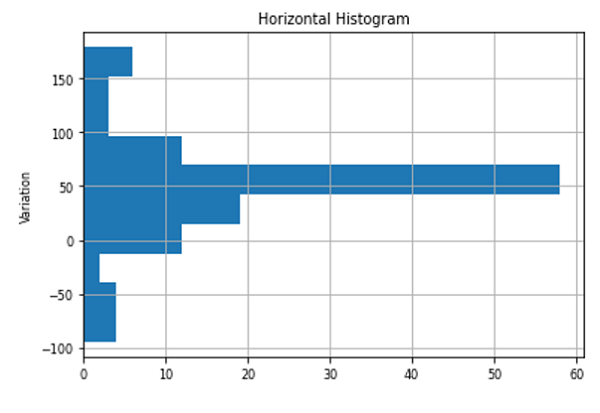# Horizontal Histogram in Python using Matplotlib

In this article, we are going to learn to plot horizontal histogram as a technique used for data visualization.
Submitted by Anuj Singh, on August 08, 2020

A histogram is a graphical technique or a type of data representation using bars of different heights such that each bar group's numbers into ranges (bins or buckets). Taller the bar higher the data falls in that bin. Similar to the conventional histogram, there is a horizontal histogram in which bars are parallel to the x-axis. A Histogram is one of the most used techniques in data visualization and therefore, matplotlib has provided a function matplotlib.pyplot.hist(orientation='horizontal') for plotting horizontal histograms.

The following example shows an illustration of the horizontal histogram.## Python code for horizontal histogram using matplotlib

```import numpy as np
import matplotlib.pyplot as plt

# Generating Data
center = np.ones(36) * 50
flier_high = np.random.rand(12) * 100 + 100
flier_low = np.random.rand(10) * -100
data = np.concatenate((spread, center, flier_high, flier_low))

plt.figure()
plt.title('Horizontal Histogram')
plt.hist(data, orientation='horizontal')
plt.ylabel('Variation')
plt.grid()
plt.figure()
```

Output:

```Output is as Figure
```